Mathematical and Physical Journal
for High Schools
Issued by the MATFUND Foundation
 Already signed up? New to KöMaL?

# KöMaL Problems in Physics, November 2005

Show/hide problems of signs:## Problems with sign 'M'

Deadline expired on December 12, 2005.

M. 264. Let us unplug a tungsten filament bedside lamp, which was on for a long time and measure the resistance of the cooling filament as a function of the elapsed time. How long does it take the resistance to decrease to half of the value of the resistance of the filament at the operating temperature.

(6 pont)

statistics## Problems with sign 'P'

Deadline expired on December 12, 2005.

P. 3834. Electric toothbrushes are becoming more and more popular. Calculate the cost of operating one during a year if it dissipates a power of 2 W, and each day you spend 4 minutes cleaning your teeth. The price of 1 kWh electric energy is 34 forints.

(3 pont)

statistics

P. 3835. In our car both the head and the rear lights are connected to a 12 V rechargeable battery through a single 30 A fuse. The two headlights are each rated 55 W, and the two deadlights are each rated 30 W, and one of them goes wrong, while the fuse melts. We have spare bulbs, but the spare fuse that we have is rated 20 A. Can we use it?

(3 pont)

statistics

P. 3836. From a 5 m high hill a 6 kg sleigh slides down, and stops somewhere on the horizontal ground. Find the work done while the sleigh is pulled back from this position to the top of the hill.

(4 pont)

statistics

P. 3837. A wooden planck of mass m stays at rest on a horizontal surface (see the figure). A pointlike object of mass m moves along the horizontal surface with a speed of, and slides onto the circular part of the planck, than flies off (friction is negligible).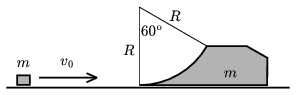a) Find the velocity of the planck after the pointlike object left it.

b) Find the greatest height to which the pointlike object ascends.

(5 pont)

statistics

P. 3838. A 3.1030kg star and a 6.1030kg star revolve about their common centre of mass. The distance between them is always 9.109m. Find the period of the motion of the stars.

(4 pont)

statistics

P. 3839. A small test tube is placed into a high cylinder which is nearly totally filled with water. The test tube is placed upside down into the water, and in its top a certain amount of air remains, such that the test tube floats. The cylinder is closed by a piece of thin rubber. If the rubber is pressed, the test tube descends and if the excess pressure on the rubber is ceased the test tube ascends. But it comes up only if it did not go below the depth of 2 m. Find the height of the that part of the test tube which was above the water before the cylinder was closed by the piece of rubber. (The mass of the cylinder-shaped test tube is 0.5 g, its inner cross section is 0.5 cm2, and its length is 5 cm. The density of water is 1000 kg/m3, the density of glass is 2500 kg/m3, and the external pressure is 105 Pa.)

(5 pont)

statistics

P. 3840. A sample of gas was compressed to a volume of 20 l adiabatically so the gas did not release or absorbe heat. The initial pressure was 101.3 kPa and was increased by 76.4% during the process. a) Calculate the change in the energy of the gas. b) Find the change of the entropy of the gas. c) By what factor did the absolute temperature of the gas increased?

(4 pont)

statistics

P. 3841. In the circuit shown in the figure switch K is turned off for a long time, then it is turned on for a long time. How does the charge of the condenser change?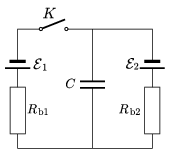Data: C=10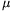F,, Rb1=0.2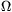,, Rb2=0.4.

(4 pont)

statistics

P. 3842. The rear-view window of a car can be rotated by a small pin, and after this rotation the image is much fainter. Thus the headlight of a car behind us will not blind the driver of our car. How is the glass of the mirror formed?

(4 pont)

statistics

P. 3843. A small pearl can move without friction along a circular rigid ring. the radius of the ring is R. Find the possible position of the pearl on the ring if the ring is rotated with an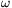angular velocity about its vertical diameter. Examine the stability of the pearl.

(5 pont)

statistics